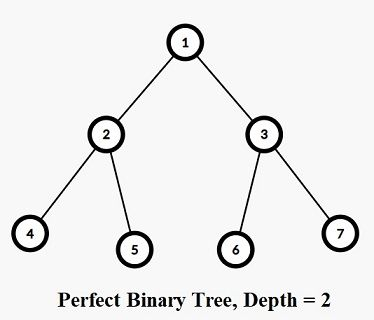# A Perfect Binary TreefsshakkhorCriterion 2020 Round 3
Limits 5s, 512 MB

A perfect binary tree is a binary tree in which all interior nodes have two children and all leaves have the same depth or same level. A perfect binary tree of level H has a total of 2H+1-1 nodes and the last level contains 2H nodes.Zakaria and Shahed have a perfect binary tree of level H containing 2H+1-1 nodes.
The root of the tree is node 1 and for each node i, its parent is $\lfloor \dfrac{i}{2} \rfloor$.

Each node i has a color Ci assigned to it.

Now you need to answer some queries.

Zakaria will select a node randomly from the subtree of X. Each node in the subtree has equal probability to get selected.

Shahed will select a node randomly from the subtree of Y. Each node in the subtree has equal probability to get selected.

What is the probability that both of the selected nodes will have the same color? The probability can be expressed as an irreducible fraction $\dfrac{A}{B}$, where A and B are non-negative integers. You need to print the value of A and B.

## Input

The first line contains an integer H (1 ≤ H ≤ 17), denoting the height of the perfect binary tree.

The next line contains 2H+1-1 positive integers where the color of the ith (1 ≤ i ≤ 2H+1-1) node is denoted by Ci (1 ≤ Ci ≤ 100000).

The next line contains an integer Q (1 ≤ Q ≤ 300000).

Each of the next Q lines contain two integers X (1 ≤ X ≤ 2H+1-1) and Y (1 ≤ Y ≤ 2H+1-1).

## Output

For each query, print the value of A and B.

## Sample

InputOutput
2
1 2 2 2 3 1 2
3
2 3
5 3
4 3

4 9
0 1
2 3HeavyLightDecompositionuDebug

### Submit